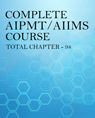Click to Chat

1800-1023-196

+91-120-4616500

CART 0

• 0

MY CART (5)

Use Coupon: CART20 and get 20% off on all online Study Material

ITEM
DETAILS
MRP
DISCOUNT
FINAL PRICE
Total Price: Rs.

There are no items in this cart.
Continue Shopping
`        In the equilibrium SO2Cl2(g) - SO2(g) +Cl2(g) at 2000K and 10 atm pressure, %Cl2 = %SO2 = 40 (by volume)then calculate Kp ?`
one year ago

```							Dear student SO2Cl2 = SO2 + Cl2100               0           020                  40       402 atm             4 atm   4 atmnow calculate Kp you will get answer as Kp = 8
```
one year ago
```							volume percent mean given mole percent thats mean it is mole fraction  cl2 mole fraction is then =.4 then partial pressure =mole fraction  *  total pressure =.4*10=4 same for so2 for so2cl2 at eq P - 4 becoz we take so2cl2 initial partial pressure p  now P -4+4+4 should equal to 10here P =6 then at eq P -4 =2  now kp =4*4/2 =8
```
5 months ago
Think You Can Provide A Better Answer ?

## Other Related Questions on AIPMT

View all Questions »### Course Features

• 731 Video Lectures
• Revision Notes
• Previous Year Papers
• Mind Map
• Study Planner
• NCERT Solutions
• Discussion Forum
• Test paper with Video Solution### Course Features

• 728 Video Lectures
• Revision Notes
• Test paper with Video Solution
• Mind Map
• Study Planner
• NCERT Solutions
• Discussion Forum
• Previous Year Exam Questions